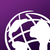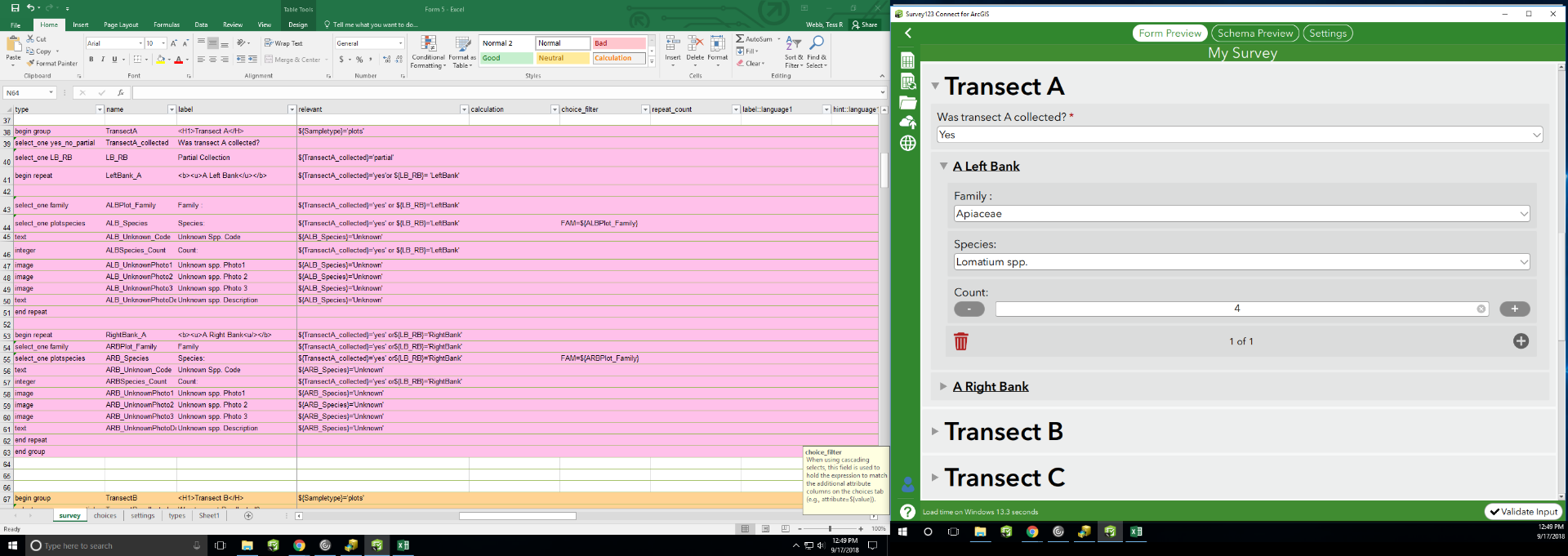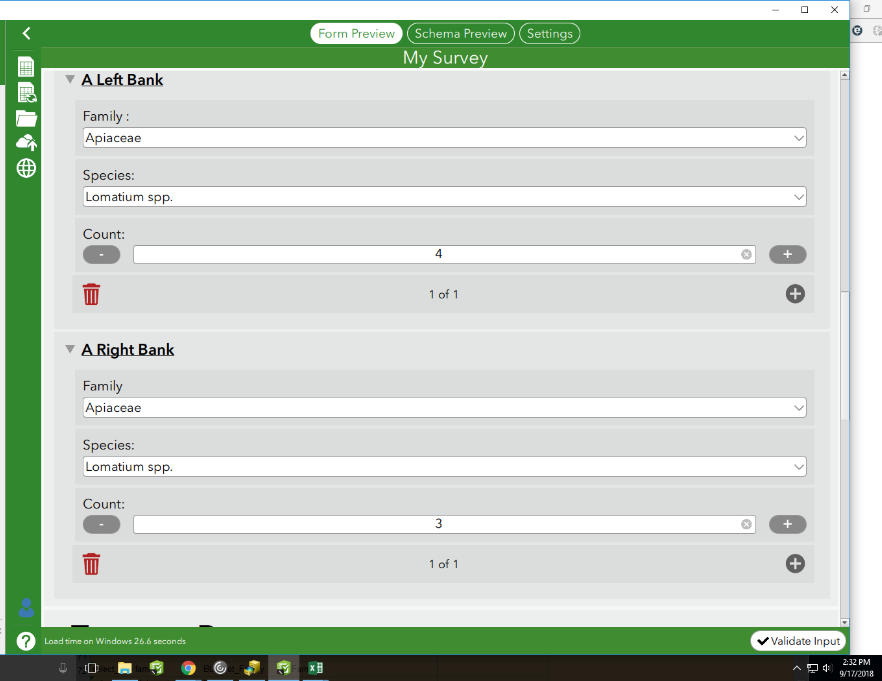Summarizing repeats while connecting a two part question?

804
6
09-17-2018 12:06 PMby
New Contributor

I am building a very complex and lengthy field form for assessing preferred forbs in riparian areas. We are collecting data at 11 transect, and at each transect two plot locations exist.. Left Bank + Right Bank.
At each plot you'll collect the family, which will then give a list of species within the family to select from, and then the count of each species observed will be collected.

I am not totally sure if this is even possible, but I am interested in having some sort of summary of each species and their associated count for each Transect, so then they can be tallied at the end.

Ie: Transect A
Lomatium spp. 6
Erysimum spp. 4

Transect B
Lomatium spp. 3

Erysimum spp. 6

Then at the end I can have a summary of all species collected in each plot to say:

Lomatium spp. 9

Erysimum spp. 10

Summarizing these can give me the count of my answers, which is helpful but counts Lomatium spp. in one plot and Lomatium spp. in another plot as two instead of 1.

I have tried using a note + sum calculation to list the species observed in one plot which is kind of helpful but I really need their associated counts as well.

If anyone knows of a round about solution for this, or if it is far to complex for the capability of Survey123 please let me know! Even if there is a script I could run the form through on the back end before submitting??

Any thoughts or suggestions would be appreciated.
I have attached a screenshot below.6 RepliesbyEsri Esteemed Contributor

Hi Tess,

This could be done with a set of calculate questions that create tally mark based on the value; for example the one for Lomatium spp. for Transect A, Left Bank would be:

if(\${ALB_species} = 'lomatium', 1, 0)

This would go immediately below the species selection question (in the same repeat).  You would then use the sum() function on that question to add the tally up.

Attached is an exampleby
New Contributor

Thanks for this James!

This would create a tally based on the number of times (for example) Lomatium was recorded, which is helpful for coding. But in addition to calculating how many time lomatium was recorded I'm also interested in the count of lomatium species observed in a plot.

So you'd record lomatium was observed and then 5 or however many plants were in the plot.

So the calculation you have suggested would list how many times Lomatium was recorded, but then is there a way to total number of lomatium plants observed in all of the plots?

So for example. The screenshot below shows 4 Lomatium in the first plot and then 3 Lomatium in the second plot.
So, I'm then looking for a way for the summary to show 7 Lomatium.

Let me know what you think??

Thanks!

TessbyEsri Esteemed Contributor

Hi Tess,

Instead of having a static 1 value in the if() function, I would use the tally question.by
New Contributor

The tally question is helpful, but the way the protocol works for our sampling, is counting each lomatium (or one of the other plants from a list of 70 species) that is observed in a plot.

For field functionality it makes sense to have a count spinner to tap as you see each lomatium to keep a running total.

Given the tally question you suggested above you would have to select the family, then the species in a repeat question over and over again to count the number of lomatium in a plot, which could show the running total as a tally. But, then the 70 other species from the list would have to be entered as a calculation for each plot which could get kind of messy.

Any thoughts on the functionality of summarizing the species (and their counts that were recorded with a spinner) for all plots combined? If its at all possible?byEsri Esteemed Contributor

Hi Tess,

I meant using your tally question as the value instead of the 1 value in the formula I provided (if that wasn't unclear):

if(\${ALB_species} = 'lomatium', \${ALBSpecies_Count}, 0)

I agree it would become a bit messy over 70 species, but this would be the case regardless if you're wanting to display it in the from (as opposed to afterwards through some table processing).by
New Contributor

Oh great, I misunderstood your last response. I will give this a shot. Thanks so much for your help!